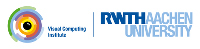OpenMesh
Using standard properties

This example shows:

• How to add and remove a standard property,
• How to get and set the value of a standard property.

As we already have seen, we can bind additional data to the mesh entities by means of properties. OpenMesh provides a set of so-called standard properties. Unlike the custom properties these have some special features and a different interface, which are the matter in this tutorial.

The following table lists all available standard properties and the suitable entity for which it can be used.

 Vertex Face Edge Halfedge Color X X X Normal X X X Position (*) X Status X X X X TexCoord X X

To add a standard property to an entity simply use the appropriate request method, e.g. `request_face_normals()`. The only exception is the position (*). It cannot be added because it is permanently available, hence it cannot be removed as well.

In this example we

1. add vertex normals to a mesh object
3. check if the file provides vertex normals and calculate them if not
4. move every vertex one unit length along its normal direction
5. print the resulting positions to std::cout

mesh.request_vertex_normals();

In a similar manner we can request the other standard properties. For example the face normals:

mesh.request_face_normals();

We need them to calculate the vertex normals with `update_normals()`, if the file didn't provide any.

But we can do more with standard properties. We can verify if the mesh has already the property vertex normals

if (!mesh.has_vertex_normals())
{
std::cerr << "ERROR: Standard vertex property 'Normals' not available!\n";
return 1;
}

And after usage we remove them again

mesh.release_vertex_normals();

But, what happens if for example the vertex status property has been requested twice? Then the first release does nothing, but the second one will remove it. The standard properties have a reference counter, which is incremented by one for each request and decremented by one for each release. If the counter reaches 0 the property will be removed from memory.

As we have seen in the table above, we have 9 dynamically requestable properties. The request functions as defined in OpenMesh::Concepts::KernelT are:

• request_edge_status()
• request_edge_colors()
• request_face_colors()
• request_face_normals()
• request_face_status()
• request_face_texture_index()
• request_halfedge_status()
• request_halfedge_normals()
• request_halfedge_texcoords1D()
• request_halfedge_texcoords2D()
• request_halfedge_texcoords3D()
• request_vertex_colors()
• request_vertex_normals()
• request_vertex_status()
• request_vertex_texcoords1D()
• request_vertex_texcoords2D()
• request_vertex_texcoords3D()

Added properties can be released by the following functions:

• release_edge_status()
• release_edge_colors()
• release_face_colors()
• release_face_normals()
• release_face_status()
• release_face_texture_index()
• release_halfedge_status()
• release_halfedge_normals()
• release_halfedge_texcoords1D()
• release_halfedge_texcoords2D()
• release_halfedge_texcoords3D()
• release_vertex_colors()
• release_vertex_normals()
• release_vertex_status()
• release_vertex_texcoords1D()
• release_vertex_texcoords2D()
• release_vertex_texcoords3D()

A property's existance can be tested with

• has_edge_status()
• has_edge_colors()
• has_face_colors()
• has_face_normals()
• has_face_status()
• has_face_texture_index()
• has_halfedge_status()
• has_halfedge_normals()
• has_halfedge_texcoords1D()
• has_halfedge_texcoords2D()
• has_halfedge_texcoords3D()
• has_vertex_colors()
• has_vertex_normals()
• has_vertex_status()
• has_vertex_texcoords1D()
• has_vertex_texcoords2D()
• has_vertex_texcoords3D()

which return true if a property has been requested before and is available.

The status property is used for marking geometry elements i.e. as selected or deleted. See Deleting geometry elements for further information.

Now we know how to add and remove standard properties, but how do we access them? Again we need the mesh object. Unlike the custom properties, where we accessed one with the mesh member function `property()`, for each standard property the mesh provides a get and a set method. We have used one pair of get/set methods already in the previous three tutorials, where we computed a new location for the vertex position. Here we move all vertices a unit length along their normal direction:

for (MyMesh::VertexIter v_it = mesh.vertices_begin();
v_it != mesh.vertices_end(); ++v_it)
{
mesh.set_point( *v_it, mesh.point(*v_it)+mesh.normal(*v_it) );
}

The get-methods take an entity handle and return the value of the desired property, and the set-methods require an additional parameter to pass the new value to the property. According to the table not every pair of get/set-methods apply to every entity. For example a face has normally no texture coordinates, hence a call to `mesh.texcoord2D( _face_handle )` will result in an error when compiling the code.

Since we know how to add/remove/access standard properties, one further question remains. What data types do they have? And are there more hidden secrets? The next tutorial (Using mesh attributes and traits) will give the answer.

The complete source looks like this:

#include <iostream>
// --------------------
#include <OpenMesh/Core/IO/MeshIO.hh>
#include <OpenMesh/Core/Mesh/TriMesh_ArrayKernelT.hh>
int main(int argc, char **argv)
{
MyMesh mesh;
if (argc!=2)
{
std::cerr << "Usage: " << argv << " <input>\n";
return 1;
}
// request vertex normals, so the mesh reader can use normal information
// if available
mesh.request_vertex_normals();
// assure we have vertex normals
if (!mesh.has_vertex_normals())
{
std::cerr << "ERROR: Standard vertex property 'Normals' not available!\n";
return 1;
}
{
std::cerr << "Error loading mesh from file " << argv << std::endl;
return 1;
}
// If the file did not provide vertex normals, then calculate them
{
// we need face normals to update the vertex normals
mesh.request_face_normals();
// let the mesh update the normals
// dispose the face normals, as we don't need them anymore
mesh.release_face_normals();
}
// move all vertices one unit length along it's normal direction
for (MyMesh::VertexIter v_it = mesh.vertices_begin();
v_it != mesh.vertices_end(); ++v_it)
{
std::cout << "Vertex #" << *v_it << ": " << mesh.point( *v_it );
mesh.set_point( *v_it, mesh.point(*v_it)+mesh.normal(*v_it) );
std::cout << " moved to " << mesh.point( *v_it ) << std::endl;
}
// don't need the normals anymore? Remove them!
mesh.release_vertex_normals();
// just check if it really works
if (mesh.has_vertex_normals())
{
std::cerr << "Ouch! ERROR! Shouldn't have any vertex normals anymore!\n";
return 1;
}
return 0;
}

Project OpenMesh, ©  Computer Graphics Group, RWTH Aachen. Documentation generated using doxygen .